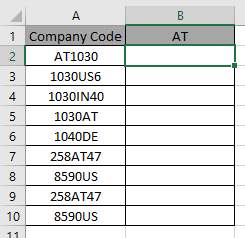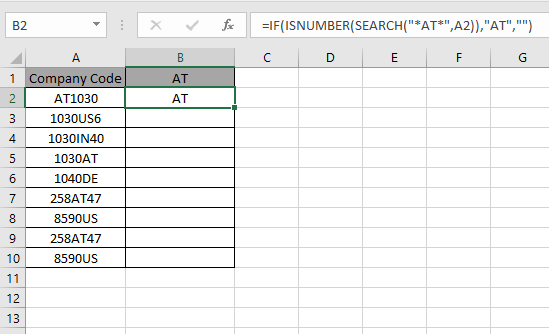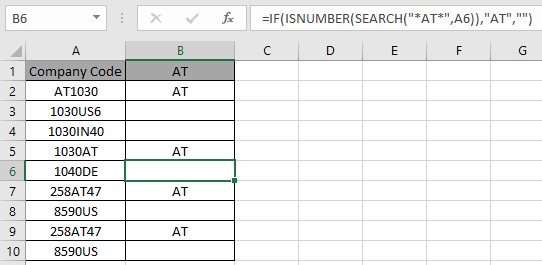# IF function with Wildcards

IF function is logic test excel function. IF function tests a statement and returns values based on the result.
Syntax:

=IF(logic_test, value_if_true, value_if_false)

We will be using the below wildcards in logic test
There are three wildcard characters in Excel

1. Question mark (?) : This wildcard is used to search for any single character.
2. Asterisk (*): This wildcard is used to find any number of characters preceding or following any character.
3. Tilde (~): This wildcard is an escape character, used preceding the question mark (?) or asterisk mark (*).

Not all functions can execute Wildcards with Excel functions.
Here is a list of functions that accepts wildcards.

• AVERAGEIF, AVERAGEIFS
• COUNTIF, COUNTIFS
• SUMIF, SUMIFS
• VLOOKUP, HLOOKUP
• MATCH
• SEARCH

Let’s understand this function using it an example.Here we have company codes on one side. And we need to find the fields where phrase AT is present anywhere in the code.

IF function doesn’t support wildcards so we will be using the SEARCH function. SEARCH function returns a number if the phrase is present within the text.

Use the formula:

=IF(ISNUMBER(SEARCH("*AT*",A2)), "AT", "")

SEARCH function accepts the wildcard (*) and finds the phrase “AT”, within A2. It returns a number if SEARCH finds the phrase.
ISNUMBER function finds the number and returns TRUE.
IF function logic_test results in TRUE and FALSE and returns “AT” if True or “”(empty string) if False.As you can see the formula catches the AT in A2 cell and returns the phrase. This shows A2 cell has “AT” pharse within text.

Now use the formula in other cells to get all fields containing the phrase “AT”.As you can see we used wildcards with IF function to get the result.

Hope you understood how to use in Excel. Explore more articles on Excel cell reference function here. Please feel free to state your query or feedback for the above article.

Related Articles:

How to use the Wildcards in Excel

IF not this or that in Microsoft Excel

IF with OR Function in Excel

Popular Articles:

How to use the VLOOKUP Function in Excel

How to use the COUNTIF function in Excel 2016

How to use the SUMIF Function in Excel

1.Is there a way of returning the wildcard value rather than AT. e.g. in A2 the formula would return 1030 into cell B2

2.What is the formula for rounding a number up or down to the nearest ones based on the decimal value of a number? I want to round up if the number is .6667 or larger and down if less than .6667. ex: 10.6667 =11 or 10.6665 = 10.

•Hey Richard,

Here you have a logical issue. So It's preferred to use the IF formula. Assume the number is F7 cell. Use =IF(F7>=10.6667,ROUNDUP(F7,0),ROUNDDOWN(F7,0)) for the above mentioned problem.